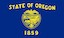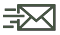An official website of the State of Oregon »

# Monthly Credit Transaction Report

HB 2017 (2017) requires DEQ, by no later than the last day of the month immediately following the month for which the calculation is completed, to calculate the volume-weighted average price of credits. The following chart represents the volume of credits traded and the average price:If you click on the graph, the spreadsheet with the underlying data is available. DEQ is also required to post the formula for the calculation.

#### Formula

The volume-weighted average price is calculated as the sum of the value (credit price * number of credits traded) of all transactions, divided by the total volume of credits traded over the month.

Volume Weighted Average Price=(∑^x▒〖(Transaction〗_x   volume*〖Transaction〗_x  price) )/(∑^x▒〖〖Transaction〗_x  volume〗)

#### ContactsSign up for email or text updates on Oregon Clean Fuels Program